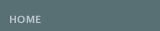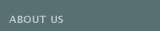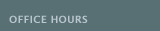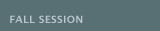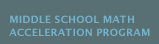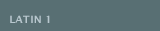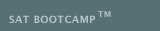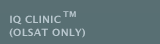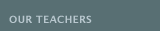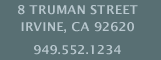The MIDDLE SCHOOL MATH ACCELERATION PROGRAM (MSMAP) at the BARUN ACADEMIC CENTER is designed to prepare the 6th grade student for the appropriate advanced math class.
 APPLIED MATH FOR THE 6TH GRADE (MSMAP) is designed for the 6th grade student for ENHANCED Math 7/8 placement in the 7th grade. This group class is aligned with the California's COMMON CORE State Standards: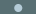Ratios and Proportional Relationships: Use ratio and rate reasoning to solve real-world and mathematical problems (by reasoning about tables of equivalent ratios, tape diagrams, double number line diagrams, or equations).The Number System: Fluently add, subtract, and divide multi-digit numbers and decimals using the standard algorithm for each operation. Understand ordering and absolute value of rational numbers. Solve real-world and mathematical problems by graphing points in all four quadrants of the coordinate plane.Expressions and Equations: Evaluate expressions at specific values of their variables. Reason about and solve one-variable equations and inequalities. Represent and analyze quantitative relationships between dependent and independent variables.Geometry: Solve real-world and mathematical problems involving area, surface area, and volume.Statistics and Probability: Develop understanding of statistical variability. Display numerical data in plots on a number line, including dot plots, histograms, and box plots.
 Students will be required to attend twice a week (one lesson to emphasize problem solving and word problems and the other to reinforce accuracy). Students are expected to complete additional exercise problem sets for homework. Students can expect homework, quizzes, a mid-term and a final. Grades will be given.
 All sixth graders will be taking the Middle SchoolMath Acceleration Test in the Spring.
 M60A Applied Math 6 (ENHANCED MATH 7/8 MSMAP)
 August 21–January 26 (23 weeks)* *September 4 (Labor Day Observed) *October 16 (Indigenous Peoples' Day Observed) *November 20–26 (Thanksgiving Recess) *December 25–January 7 (Winter Recess) *January 15 (Martin Luther King Day Observed)
 Mondays 4:00–6:00pm CHAALAN
 October 21–January 27 (8 weeks)* *November 20–26 (Thanksgiving Recess) *December 25–January 7 (Winter Recess)
 Saturday, October 21 9:00–11:00am Saturday, October 28 9:00–11:00am Saturday, November 4 9:00–11:00am Saturday, November 11 9:00–11:00am Saturday, December 2 9:00–11:00am Saturday, December 9 9:00–11:00am Saturday, January 20 9:00–11:00am Saturday, January 27 9:00–11:00am

 The MIDDLE SCHOOL MATH ACCELERATION PROGRAM (MSMAP) at the BARUN ACADEMIC CENTER is designed to prepare the 6th grade student for the appropriate advanced math class.
 MATH 7 (MSMAP) is designed for the select 6th grade student for MATH 1 placement in the 7th grade. This group class is aligned with the California's COMMON CORE State Standards:Ratios and Proportional Relationships: Use proportional relationships to solve multistep ratio and percent problems.Expressions and Equations: Use variables to represent quantities in a real-world or mathematical problem, and construct equations and inequalities to solve problems by reasoning about the quantities.Geometry: Use facts about supplementary, complementary, vertical, and adjacent angles in a multi-step problem to write and solve equations for an unknown angle in a figure. Solve real-world problems involving area, volume and surface area of 2 and 3 dimensional objects composed of triangles, quadrilaterals, polygons, cubes, and right prisms.Statistics and Probability: Investigate chance processes and develop, use, and evaluate probability models. Develop a probability model and use it to find probabilities of events.
 Students will be required to attend twice a week (one lesson to emphasize problem solving and word problems and the other to reinforce accuracy). Students are expected to complete additional exercise problem sets for homework. Students can expect homework, quizzes, a mid-term and a final. Grades will be given.
 All sixth graders must first pass the Middle School Math Acceleration Testto take the Math 1 Acceleration Test in the Spring.
 M00A Math 7 (Math 1 Placement-MSMAP)
 August 21–January 26 (23 weeks)* *November 20–26 (Thanksgiving Recess) *December 25–January 7 (Winter Recess)
 Thursdays 4:00–6:00pm CHAALAN
 October 21–January 27 (8 weeks)* *November 20–26 (Thanksgiving Recess) *December 25–January 7 (Winter Recess)
 Saturday, October 21 9:00–11:00am Saturday, October 28 9:00–11:00am Saturday, November 4 9:00–11:00am Saturday, November 11 9:00–11:00am Saturday, December 2 9:00–11:00am Saturday, December 9 9:00–11:00am Saturday, January 20 9:00–11:00am Saturday, January 27 9:00–11:00am